# GSEB Solutions Class 6 Maths Chapter 14 Practical Geometry Ex 14.6

Gujarat Board GSEB Textbook Solutions Class 6 Maths Chapter 14 Practical Geometry Ex 14.6 Textbook Questions and Answers.

## Gujarat Board Textbook Solutions Class 6 Maths Chapter 14 Practical Geometry Ex 14.6

Question 1.
Draw ∠POQ of measure 75° and find its line of symmetry.
Solution:
Steps of construction:
Step I: Construct BOA = 90°, where ∠AOQ = 60° and ∠BOQ = 30°.
Step II: Draw $$\overrightarrow{\mathrm{OP}}$$, the angle bisector of ∠BOQ, such that ∠QOP = $$\frac { 1 }{ 2 }$$ ∠BOQ.
∠QOP = $$\frac { 1 }{ 2 }$$ (30°) = 15°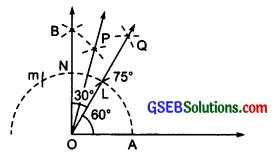Step III: Since, 60° + 15° = 75°
So, ∠AOQ + ∠QOP = ∠POA Thus, ∠POA = 75°.

Question 2.
Draw an angle of measure 147° and construct its bisector.
Solution:
Steps of construction:
Step I: Draw a ray $$\overrightarrow{\mathrm{AB}}$$.
Step II: Using a protractor construct ∠BAC = 147°.
Step III: With centre ‘A’ and a convenient radius, draw an arc which intersects the arms $$\overrightarrow{\mathrm{AB}}$$ and $$\overrightarrow{\mathrm{AC}}$$ at P and Q respectively.Step IV: With centre P and radius more than half of PQ, draw an arc.
Step V: With centre Q and keeping the same radius, draw another arc that intersects the previous arc at R.
Step VI: Join A and R. Produce AR.
Thus, $$\overrightarrow{\mathrm{AR}}$$ is the required angle bisector of ∠BAC.Question 3.
Draw a right angle and construct its bisector.
Solution:
Steps of construction:
Step I: Draw a line l and mark a point ‘O’ on it.
Step II: With centre ‘O’ and a convenient radius, draw an arc which intersects l at A and B.
Step III: With centres at A and B and radius more than half of AB, draw two arcs which intersect each other at C.Step IV: Join OC, such that ∠AOC is a right angle.
Step V: A and D as centres and radius more than half of AD, draw two arcs which cut each other at E.
Step VI: Join OE and produce it.
Thus, $$\overrightarrow{\mathrm{OE}}$$ is the required angle bisector of right angle ∠AOC, i.e. $$\overrightarrow{\mathrm{OE}}$$ is the bisector of the right angle.

Question 4.
Draw an angle of measure 153° and divide it into four equal parts.
Solution:
Steps of construction:
Step I: Draw a ray $$\overrightarrow{\mathrm{AB}}$$.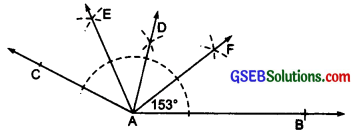Step II: Using a protractor, construct ∠BAC = 153°.
Step III: Draw $$\overrightarrow{\mathrm{AD}}$$, bisector of ∠BAC.
Step IV: Again, draw $$\overrightarrow{\mathrm{AE}}$$, bisector of ∠DAC.
Step V: Also, draw $$\overrightarrow{\mathrm{AF}}$$, bisector of ∠BAD.
Thus, $$\overrightarrow{\mathrm{AE}}$$, $$\overrightarrow{\mathrm{AD}}$$ and $$\overrightarrow{\mathrm{AF}}$$, divide the given ∠BAC into four equal parts.Question 5.
Construct with ruler and compasses, angles of following measures:
(a) 60°
Solution:
In figure ∠AOB = 60°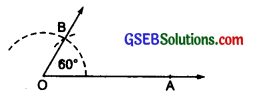(b) 30°
Solution:
∵ $$\frac { 1 }{ 2 }$$ (60°) = 30°∴ In the figure, ∠AOC = 30°

(c) 90°
Solution:
In the figure, ∠POQ = 90°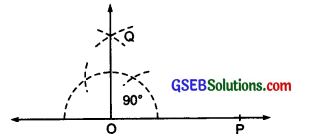(d) 120°
Solution:
Since 2 × (60°) = 120°
∴ In the figure, ∠AOC = 120°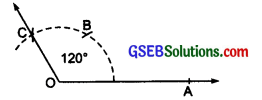(e) 45°
Solution:
∵ $$\frac { 1 }{ 2 }$$ (90°) = 45°
∴ In the figure, ∠POR = 45°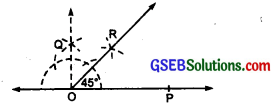(f) 135°
Solution:
Since, 90° + 45° = 135°
i.e. 90° + $$\frac { 1 }{ 2 }$$(90°) = 135°
.’. In the figure, ∠AOD = 135°.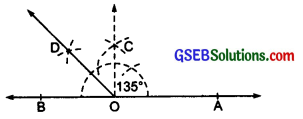Question 6.
Draw an angle of measure 45° and bisect it.
Solution:
Steps of construction:
Step I: Draw a ray OA.
Step II: Construct ∠AOQ = 45°.
Step III: With centre C and radius more than CD, draw an arc.Step IV: With the same radius and centre at D, draw another arc which intersects the arc at B.
Step V: Join OB and produce it.
Thus, $$\overrightarrow{\mathrm{OB}}$$, bisects ∠AOQ into two equal parts.
i.e. ∠AOB = $$\frac { 1 }{ 2 }$$(45°) =22$$\frac{1^{\circ}}{2}$$Question 7.
Draw an angle of measure 135° and bisect it.
Solution:
Steps of construction:
Step I: Draw a line AB and mark a point O on it.
Step II: Construct ∠AOC = 135°.
Step III: Draw $$\overrightarrow{\mathrm{OD}}$$, bisector ∠AOC.
Thus, ∠AOD = $$\frac { 1 }{ 2 }$$ (135°) = 67$$\frac{1^{\circ}}{2}$$Question 8.
Draw an angle of 70°. Make a copy of it using only a straight edge and compasses.
Solution:
Steps of construction:
Step I: Draw a line 1 and mark a point O on it.
Step II: Using a protractor construct ∠AOB = 70°.
Step III: With centre O and a suitable radius, draw an arc which intersects $$\overrightarrow{\mathrm{OA}}$$ and $$\overrightarrow{\mathrm{OB}}$$ at E and F respectively.Step IV: Draw a ray $$\overrightarrow{\mathrm{PQ}}$$.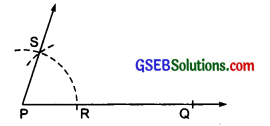Step V: Keeping the same radius and centre P, draw an arc intersecting $$\overrightarrow{\mathrm{PQ}}$$ at R.
Step VI: With centre R and radius equal to EF, draw an arc intersecting the previous arc at S.
Step VII: Join PS and produce it.
Thus, ∠QPS is a copy of ∠AOB = 70°.

Question 9.
Draw an angle of 40°. Copy its supplementary angle.
Solution:
Steps of construction:
Step I: By using protractor draw ∠AOB = 40°.
∠COF is the supplementary angle.
Step II: With centre O and a convenient radius, draw an arc which intersects $$\overrightarrow{\mathrm{OC}}$$ and $$\overrightarrow{\mathrm{OB}}$$ at E and F respectively.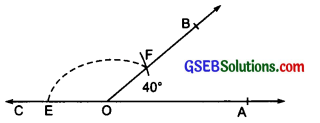Step III: Draw a ray $$\overrightarrow{\mathrm{QR}}$$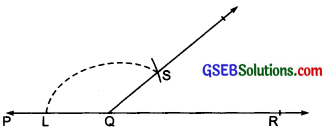Step IV: With centre Q and same radius, draw an arc intersecting $$\overrightarrow{\mathrm{PQ}}$$ at L.
Step V: With centre L and radius equal to EF draw an arc which intersects the previous arc at S.
Step VI: Join QS and produce it.
Thus, ∠PQS is the copy of the supplementary angle ∠COB